# pi Network in Network Analysis

## π or pi Network in Network Analysis:

Consider following two port ’π’ or pi Network type network with assumed voltages V1 and V2 and current I1 end I2 as shown in Fig. 6.11.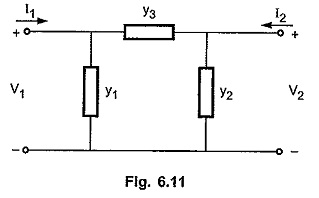Let the y-parameters of the above network are represented as follows,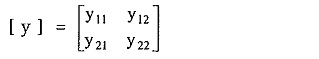We know that, y-parameter for any network can be calculated by short circuiting input and output ports ie by making V1 = 0 and V2 = 0.

With the port 2-2′ short circuited :

We have V2 = 0

Hence, y2 will be directly shorted.

Total admittance looking into network from port 1-1′ is equal to (y1 + y3).

Hence, current I1 in port 1-1′ can be calculated as follows,The current I2 in port 2-2′ can be calculated as follows.

Note that current I2 will be negative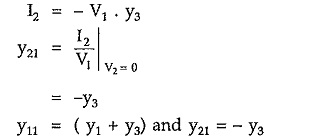With the port 1-1′ short circuited :

We have V1 = 0. Hence, y1 will be directly shorted. Total admittance looking back into network from port 2-2′ is equal to (y2 + y3).

Hence, current I2 in port 2-2′ can be calculated as follows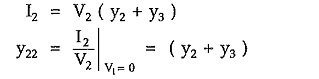The current I1 in port 1-1′ can be calculated as follows.

Note that current I1 will be negative.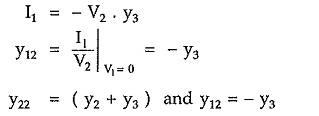Consider the expression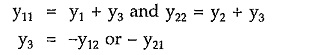substituting y3 = -y12 in first two expressions, we have,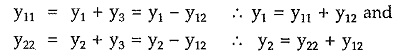Hence, the circuit elements of the equivalent ’π’ of pi network are as follows,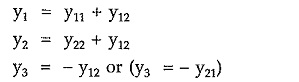Updated: November 6, 2019 — 5:54 am